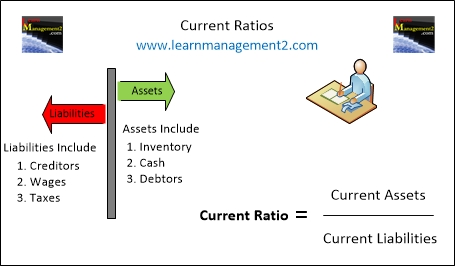# Liquidity Ratios

## Current Ratio (Working Capital Ratio)

Financial Ratios assess how well a business is doing, they usually involve comparing performance against the amount of money invested in the business. Liquidity ratios examine whether a business has enough money to pay the money it owes.

In this article we look at how to calculate the current ratio, which is one of two liquidity ratios; the other liquidity ratio is called the Acid Test Ratio.This diagram shows how to calculate the Liquidity Ratio called the Current Ratio

### How Do You Calculate The Current Ratio?

The current ratio is sometimes called the working capital ratio. It is calculated by dividing current assets by current liabilities.

Current Ratio              Current Assets
=          _________

Current Liabilities

### Example

For example if my current assets are £4000 and my current liabilities are £2000 I would work out my current ratio as follows

Current Assets                        4000
_________                  =          _________      = 2

Current Liabilities                    2000

### How to write Liquidity Ratios

As Liquidity ratios are expressed against 1, the ratio for the example above is 2:1. This means that in the example there is enough money to pay the liabilities twice.

### Why Does the Current Ratio Use Current Assets in the Calculation

Current assets are used in this ratio because they can be converted into cash fairly easily. Current assets are things that can be converted into cash within one year. Examples of current assets are

1. Stock (Inventory)
2. Cash
3. Debtors (money owed to the business)

### Why Does the Current Ratio Use Current Assets in the Calculation

Current liabilities are used in this calculation because the current ratio is a liquidity ratio which analyse short term assets and liabilities. Current liabilities are debt that the needs to be paid within a year. Examples of current liabilities are

1. Accounts payable
2. Money owed to employees (wages payable)
3. Taxes owed (taxes payable)

### Conclusion

We hope you've enjoyed learning about the current ratio. We have related Financial Ratio articles for you to read; they are a great way to increase your understanding about Financial Ratios.

Related Articles: | Current Ratio | Liquidity Ratios Overview | Liquidity & Working Capital | Introduction To Financial Ratios